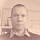# Data Science: Computation of Eigenvectors — Gershgorin circles.

This story is part of my Data Science series.

In this article we restrict our attention to the problem of finding eigenvector/eigenvalue pairs for a finite dimensional linear operator.

In many applications of machine learning one is interested in those eigenvectors that belong to the highest eigenvalues in absolute value. One very prominent example is principle component analysis (see here).

A simple criterion to get an idea where these eigenvalues are located are the Gershgorin circles.

## Gershgorin circles:

To get an idea how to apply this, let us compute the Gershgorin circles of the covariance matrix of the feature data from the data obtained from here.

The implementation will be done in Rust.## An Introduction into RustSince the covariance matrix is symmetric, all the eigenvalues are on the real axis. This considerably facilitates the usage of the result.

The dependencies will be like this, that is, using `ndarray` utilities:

`[dependencies]ndarray = "0.15.0"ndarray-csv = "0.5.1"`

`use csv::ReaderBuilder;use ndarray::{Array2, Axis};use ndarray_csv::Array2Reader;pub fn load_data() -> Array2<f64> {    let file = File::open("data/pci/wdbc-processed.csv").unwrap();    let mut reader = ReaderBuilder::new().has_headers(true).from_reader(file);    reader.deserialize_array2_dynamic::<f64>().unwrap()}`